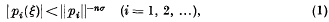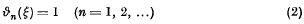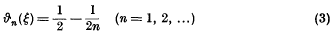Home

# The real cubic case of Mahler's conjecture

## Extract

For any (real or complex) transcendental number ξ and any integer n > 0 let ϑn(ξ) be the least upper bound of the set of all positive numbers σ for which there exist infinitely many polynomials p1(x), p2(x), … of degree n, with integer coefficients, satisfyingwhere ‖pi‖ denotes the “height” of pi(x), i.e. the maximum modulus of the coefficients. Plainly ϑn(ξ) serves as a measure of how well (or how badly) the number zero can be approximated by values of nth degree integral polynomials at the point ξ. It can be shown by means of the “Schubfachprinzip” that, at worst,if the transcendental number ξ is real, andif it is complex, i.e.ϑn(ξ) is never smaller than these bounds. Furthermore, a conjecture of K. Mahler may be interpreted as stating that for almost all real and for almost all complex numbers the equations (2) and (3), respectively, are actually true; in other words, almost all transcendental numbers have the worst possible approximation property for any degree n.

## References

Hide All
MathJax
MathJax is a JavaScript display engine for mathematics. For more information see http://www.mathjax.org.

# The real cubic case of Mahler's conjecture

## Metrics

### Full text viewsFull text views reflects the number of PDF downloads, PDFs sent to Google Drive, Dropbox and Kindle and HTML full text views.

Total number of HTML views: 0
Total number of PDF views: 0 *Loading metrics...

### Abstract viewsAbstract views reflect the number of visits to the article landing page.

Total abstract views: 0 *Loading metrics...

* Views captured on Cambridge Core between <date>. This data will be updated every 24 hours.

Usage data cannot currently be displayed